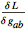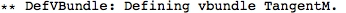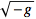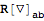xTras Tutorial

# Metric Variations

xTras automatically defines 'proper' variations with respect to the metric via VarD and VarL whenever you define a metric.
 VarD[g[-a,-b],cd][L] returnswhile integrating by parts with respect to the covariant derivative cd. VarL[g[-a,-b],cd][L] returnswhile integrating by parts with respect to the covariant derivative cd.

Computing variations w.r.t. the metric.

Let's begin with defining a manifold.DefMetric has a new option, DefMetricPerturbation. It defaults to True, and so by default a metric perturbation is defined whenever you define a metric.
 Out=In xTras, defining a metric perturbation automatically defines proper metric variations. All in all, defining a metric automatically defines proper metric variations.We can now perform variations with respect to the metric:
 Out=Using VarL automatically takes care of factors of:
 Out=Varying with respect to the inverse metric gives an overall minus sign:
 Out=Out=More complicated expressions can also be varied easily:
 Out=Out=This can be simplified further with the help of FullSimplification:
 Out=